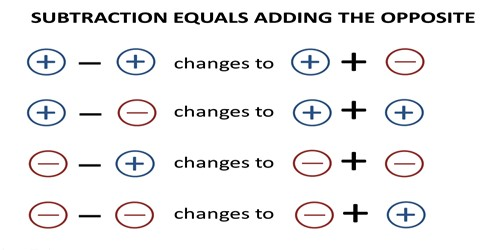Mathematic

# Integer Addition EquationsAn equation is a mathematical statement that has an expression on the left side of the equals sign (=) with the same value as the expression on the right side. An example of an equation is 2 + (- 6) = – 4.

One of the terms in an equation may not be know and needs to be determined. Often this unknown term is represented by a letter such as x. (e.g. 2 + x = – 4).

The solution of an equation is finding the value of the unknown x. To find the value of x we can use the subtractive equation property which says: “The two sides of an equation remain equal if the same number is subtracted from each side.” We may also use the additive equation property which says: “The two sides of an equation remain equal if the same number is added to each side.”

Example:

– 5 + x = 4

– 5 + x + 5 = 4 + 5

0 + x = 9

x = 9

Check the answer by substituting the answer (9) back into the equation.

– 5 + 9 = 4

Explanation:

Integer Addition equations with 3 digit integers

An equation is a mathematical statement such that the expression on the left side of the equals sign (=) has the same value as the expression on the right side. An example of an equation is 200 + (- 600) = – 400.

One of the terms in an equation may not be know and needs to be determined. Often this unknown term is represented by a letter such as x. (e.g. 200 + x = – 400).

The solution of an equation is finding the value of the unknown x. To find the value of x we can use the subtractive equation property which says: The two sides of an equation remain equal if the same number is subtracted from each side. We may also use the additive equation property which says: The two sides of an equation remain equal if the same number is added to each side.

Example:

– 500 + x = 400

– 500 + x + 500 = 400 + 500

0 + x = 900

x = 900

Check the answer by substituting the answer (900) back into the equation.

– 500 + 900 = 400

Integer Addition equations with 4 digit integers

An equation is a mathematical statement such that the expression on the left side of the equals sign (=) has the same value as the expression on the right side. An example of an equation is 2000 + (- 6000) = – 4000.

One of the terms in an equation may not be know and needs to be determined. Often this unknown term is represented by a letter such as x. (e.g. 2000 + x = – 4000).

The solution of an equation is finding the value of the unknown x. To find the value of x we can use the subtractive equation property which says: The two sides of an equation remain equal if the same number is subtracted from each side. We may also use the additive equation property which says: The two sides of an equation remain equal if the same number is added to each side.

Example:

– 5000 + x = 4000

– 5000 + x + 5000 = 4000 + 5000

0 + x = 9000

x = 9000

Check the answer by substituting the answer (9000) back into the equation.

– 5000 + 9000 = 4000

Information Source: